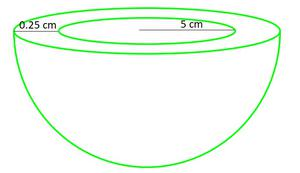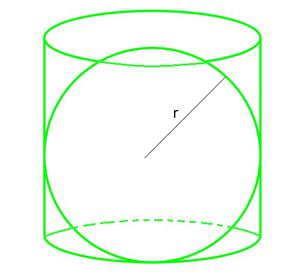# Class 9 NCERT Solutions- Chapter 13 Surface Areas And Volumes – Exercise 13.4

### Question 1. Find the surface area of a sphere of radius:

(i) 10.5cm (ii) 5.6cm (iii) 14cm

(Assume π=22/7)

Solution:

We know that, Surface area of sphere (SA) = 4πr²

(i) Radius of sphere, r = 10.5 cm

SA = 4×(22/7)×10.52 = 1386

Therefore, Surface area of sphere is 1386 cm²

(ii) Radius of sphere, r = 5.6cm

SA = 4×(22/ 7)×5.62 = 394.24

Therefore, Surface area of sphere is 394.24 cm²

(iii) Radius of sphere, r = 14cm

SA = 4×(22/7)×(14)2

= 2464

Therefore, Surface area of sphere is 2464 cm²

### Question 2. Find the surface area of a sphere of diameter:

(i) 14cm (ii) 21cm (iii) 3.5cm

(Assume π = 22/7)

Solution:

(i) Radius of sphere, r = diameter/2 = 14/2 cm = 7 cm

We know that, Surface area of sphere = 4πr²= 4×(22/7)×72 = 616

Surface area of a sphere is 616 cm²

(ii) Radius of sphere,r= diameter/2=21/2 = 10.5 cm

We know that, Surface area of sphere = 4πr²

= 4×(22/7)×10.52 = 1386

Surface area of a sphere is 1386 cm²

Therefore, the surface area of a sphere having diameter 21cm is 1386 cm²

(iii) Radius(r) of sphere = 3.5/2 = 1.75 cm

We know that, Surface area of sphere = 4πr²

= 4×(22/7)×1.752 = 38.5

Surface area of a sphere is 38.5 cm²

### Question 3. Find the total surface area of a hemisphere of radius 10 cm. [Use π=3.14]

Solution:

Given, Radius of hemisphere, r = 10cm

Formula: Total surface area of hemisphere = 3πr²

= 3×3.14×102 = 942

Therefore, total surface area of given hemisphere is 942 cm².

### Question 4. The radius of a spherical balloon increases from 7cm to 14cm as air is being pumped into it. Find the ratio of surface areas of the balloon in the two cases.

Solution:

We assume that r1 and r2 be the radii of spherical balloon and spherical balloon when air is pumped into it respectively. So,

r1 = 7cm

r2 = 14 cm

Now, Required ratio = (initial surface area)/(Surface area after pumping air into balloon)

= 4(r1)²/4(r2)²

= (r1/r2)²

= (7/14)² = (1/2)² = ¼

Therefore, the ratio between the surface areas is 1:4.

### Question 5. A hemispherical bowl made of brass has inner diameter 10.5cm. Find the cost of tin-plating it on the inside at the rate of Rs 16 per 100 cm². (Assume π = 22/7)

Solution:

Given, Inner radius of hemispherical bowl, say r = diameter/2 = (10.5)/2 cm = 5.25 cm

We know, Formula for Surface area of hemispherical bowl = 2πr²

= 2×(22/7)×(5.25)2 = 173.25cm²

Cost of tin-plating 100 cm² area = Rs 16

So, Cost of tin-plating 1 cm² area = Rs 16 /100

Cost of tin-plating 173.25 cm² area = Rs. (16×173.25)/100 = Rs 27.72

Therefore, the cost of tin-plating the inner side of the hemispherical bowl at the rate of Rs 16 per 100 cm² is Rs 27.72.

### Question 6. Find the radius of a sphere whose surface area is 154 cm². (Assume π = 22/7)

Solution:

Let the radius of the sphere be r.

Surface area of sphere = 154 (given)

So,

4πr² = 154

r² = (154×7)/(4×22) = (49/4)

r = (7/2) = 3.5cm

Therefore, the radius of the sphere is 3.5 cm.

### Question 7. The diameter of the moon is approximately one-fourth of the diameter of the earth. Find the ratio of their surface areas.

Solution:

Let the diameter of earth be d, then the diameter of moon will be d/4 (as per given statement)

So, Radius of moon = ½×d/4 = d/8

Surface area of moon = 4π(d/8)²

Surface area of earth = 4π(d/2)²

The ratio between their surface areas is 1:16.

### Question 8. A hemispherical bowl is made of steel, 0.25 cm thick. The inner radius of the bowl is 5cm. Find the outer curved surface of the bowl. (Assume π =22/7)

Solution:

Given:Inner radius of hemispherical bowl = 5cm

Thickness of the bowl = 0.25 cm

Outer radius of hemispherical bowl = (5+0.25) cm = 5.25 cm

We know, formula for outer CSA of hemispherical bowl = 2πr², where r is radius of hemisphere

= 2×(22/7)×(5.25)²= 173.25

Therefore, the outer curved surface area of the bowl is 173.25 cm².

### Question 9. A right circular cylinder just encloses a sphere of radius r (see fig.). Find

(i) surface area of the sphere,

(ii) curved surface area of the cylinder,

(iii) ratio of the areas obtained in (i) and (ii).Solution:

(i) Surface area of sphere = 4πr², where r is the radius of sphere

(ii) As Height of cylinder, h = r+r =2r

And Radius of cylinder = r

CSA of cylinder formula = 2πrh = 2πr(2r) (using value of h)

= 4πr²

(iii) Ratio between areas = (Surface area of sphere)/CSA of Cylinder)

= 4r2/4r2 = 1/1

Therefore, Ratio of the areas obtained in (i) and (ii) is 1:1.

Whether you're preparing for your first job interview or aiming to upskill in this ever-evolving tech landscape, GeeksforGeeks Courses are your key to success. We provide top-quality content at affordable prices, all geared towards accelerating your growth in a time-bound manner. Join the millions we've already empowered, and we're here to do the same for you. Don't miss out - check it out now!

Previous
Next